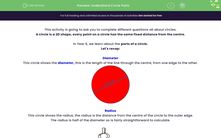# Understand Circle Parts

In this worksheet, students will be asked to find parts of a circle, the diameter, radius and circumference.Key stage:  KS 2

Curriculum topic:   Geometry: Properties of Shapes

Curriculum subtopic:   Know Circle Parts

Popular topics:   Geometry worksheets

Difficulty level:#### Worksheet Overview

This activity is going to ask you to complete different questions all about circles.

A circle is a 2D shape, every point on a circle has the same fixed distance from the centre.

In Year 6, we learn about the parts of a circle.

Let's recap:

Diameter

This circle shows the diameter, this is the length of the line through the centre, from one edge to the other.This circle shows the radius, the radius is the distance from the centre of the circle to the outer edge.

The radius is half of the diameter so is fairly straightforward to calculate.Circumference

A circle's circumference is the distance around the edge.Example

If a circle's diameter is 12cm, what is its radius?

6cm

Remember, the diameter is double the radius, so we would need to halve the diameter to get the radius.

### What is EdPlace?

We're your National Curriculum aligned online education content provider helping each child succeed in English, maths and science from year 1 to GCSE. With an EdPlace account you’ll be able to track and measure progress, helping each child achieve their best. We build confidence and attainment by personalising each child’s learning at a level that suits them.

Get started